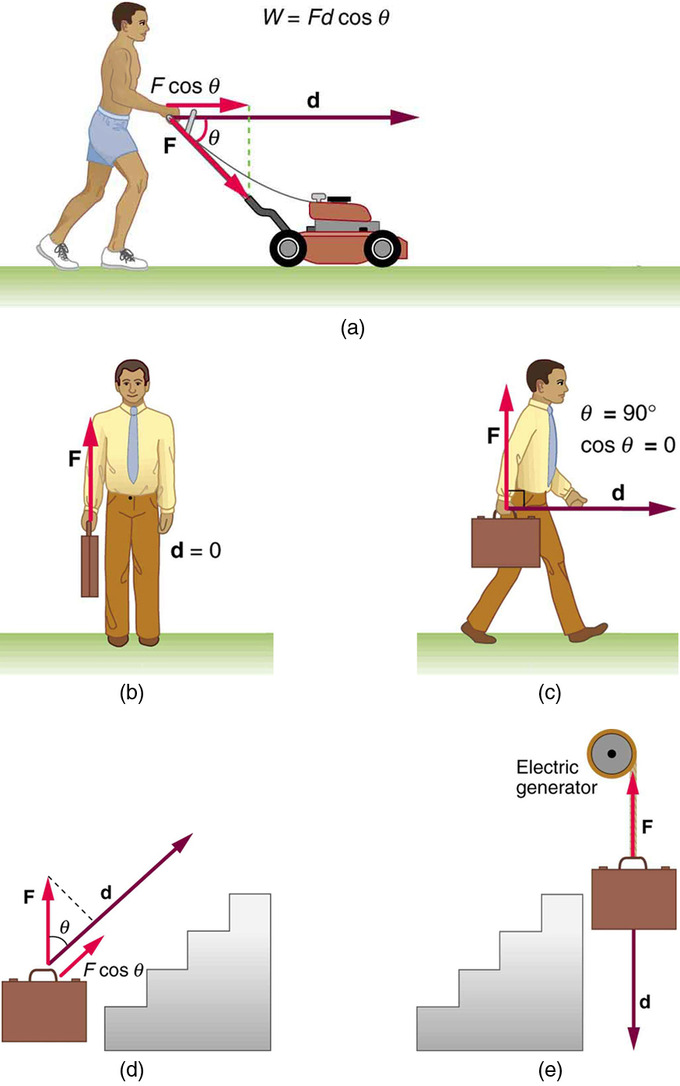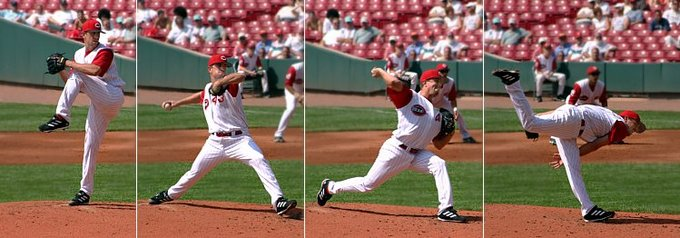## Introduction to Work and Energy

Work is the energy associated with the action of a force.

### Learning Objectives

Describe relationship between work, energy, and force

### Key Takeaways

#### Key Points

• Work is the transfer of energy by a force acting on an object as it is displaced.
• The work done by a force is zero if the displacement is either zero or perpendicular to the force.
• The work done is positive if the force and displacement have the same direction, and the work done is negative if they have opposite direction.

#### Key Terms

• energy: A quantity that denotes the ability to do work and is measured in a unit dimensioned in mass × distance²/time² (ML²/T²) or the equivalent.

The work done on a system by a constant force is the product of the component of the force in the direction of motion times the distance through which the force acts. For one-way motion in one dimension, this is expressed in equation form as W=Fdcosθ, where W is work, F is the magnitude of the force on the system, d is the magnitude of the displacement of the system, and θ is the angle between the force vector F and the displacement vector d.

Work, Power, and Energy: Biology is useful.

Take this example of work in action from: (A) The work done by the force F on this lawn mower is Fdcosθ. Note that Fcosθ is the component of the force in the direction of motion. (B) A person holding a briefcase does no work on it, because there is no motion. No energy is transferred to or from the briefcase. (C) The person moving the briefcase horizontally at a constant speed does no work on it, and transfers no energy to it. (D) Work is done on the briefcase by carrying it up stairs at constant speed, because there is necessarily a component of force F in the direction of the motion. Energy is transferred to the briefcase and could, in turn, be used to do work. (E) When the briefcase is lowered, energy is transferred out of the briefcase and into an electric generator. Here the work done on the briefcase by the generator is negative, removing energy from the briefcase, because F and d are in opposite directions.Examples of Work: This is how work in progress and energy co-exist and operate. Work is the energy associated with the action of a force.

In physics, a force is said to do work when it acts on a body so that there is a displacement of the point of application, however small, in the direction of the force. Thus a force does work when there is movement under the action of the force. The work done by a constant force of magnitude F on a point that moves a distance d in the direction of the force is the product:

$\text{W}=\text{Fd}$

For example, if a force of 10 newton (F = 10 N) acts along point that travels two meters (d = 2 m), then it does the work W = (10 N)(2 m) = 20 N m = 20 J. This is approximately the work done lifting a 1 kg weight from the ground to over a person’s head against the force of gravity. Notice that the work is doubled either by lifting twice the weight in the same distance or by lifting the same weight twice the distance.

Work is closely related to energy. The conservation of energy states that the change in total internal energy of a system equals the added heat minus the work performed by the system (see the first law of thermodynamics, and ):Baseball Pitcher: A baseball pitcher does work on a baseball by throwing the ball at some force, F, over some distance d, which for the average baseball field, is about 60 feet.

$\delta \text{E}=\delta \text{Q}-\delta \text{W}$

Also, from Newton’s second law for rigid bodies, it can be shown that work on an object is equal to the change in kinetic energy of that object:

$\text{W}=\Delta \text{KE}$

The work of forces generated by a potential function is known as potential energy and the forces are said to be conservative. Therefore work on an object moving in a conservative force field is equal to minus the change of potential energy of the object:

$\text{W}=-\Delta \text{PE}$

This shows that work is the energy associated with the action of a force, and so has the physical dimensions and units of energy.